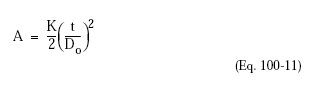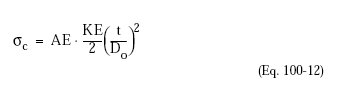﻿ Cylindrical Shells Subjected to External Pressure – Oil & Gas Industry Technology Updates

## Cylindrical Shells Subjected to External Pressure

The critical collapse pressure (Pc) of a cylindrical shell under external pressure depends on two characteristic geometric ratios: t/Do and L/Do, where t is the shell thickness, L is the unstiffened length, and Do is the outside diameter. If L is short enough, the cylinder can fail by plastic yielding in compression at a stress above the yield strength of the material, and the ordinary membrane stress equation applies. This type of failure, however, is likely only with heavy wall cylinders.

The critical strain, A, at which a thin wall cylinder under external pressure will collapse can be approximated by:where K is a factor that depends on the length-to-radius, L/R, and Do/t ratios. The critical compressive stress (Qc) corresponding to the above critical strain is approximated by:where E is the modulus of elasticity of the material.